# CellRank beyond RNA velocity¶

This tutorial shows how to work with CellRank when no RNA veloctiy information is available - either because this just isn't available at the moment, or maybe because RNA velocity does not work well in your biological system. RNA velocity is a fantastic method, but like any other method, it has its limitations. To name just one, it relies on having sufficient unspliced counts in the key biological driver genes for your system in order to reliably estimate the direction of cellular dynamics.

When no RNA velocity information is available, CellRank can still be used. You just need to work with a different kernel to compute your transition matrix. If you're unsure what a kernel is, please go through our kernels and estimators tutorial first. One possibility to get a directed cell-cell transition matrix is to use a KNN graph and a pseudotime to bias the graph such that edges are likely to point into the direction of increasing pseudotime - this idea has been explored in Palantir and is implemented in CellRank via the PseudotimeKernel. In this tutorial, we'll take a different approach that circumvents the need to define a root cell for pseudotime computation by using the CytoTRACE score to direct graph-edges to point into the direction of increasing differentiation status. In CellRank, this can be achieved using the CytoTraceKernel. We'll give more details on this later on.

As an example, we'll apply this to the axial mesoderm lineage of the zebrafish embryo data generated by Farrell et al., Science 2018. Note that the same subset of this data has been used in Supplementary Fig. 7 of Nowotschin et al., Nature 2019 to show generalization of their Harmony algorithm. We restricted data from the original study to cells labelled as Lineage_Prechordal_Plate and Lineage_Notochord.

## Import packages and data¶

In :
import sys

!pip install -q git+https://github.com/theislab/[email protected]

In :
import scvelo as scv
import scanpy as sc
import cellrank as cr
import numpy as np

scv.settings.verbosity = 3
scv.settings.set_figure_params("scvelo")
cr.settings.verbosity = 2


The following command will download the adata object (43 MB) and save it under datasets/zebrafish.h5ad.

In :
adata = cr.datasets.zebrafish()

100%|██████████| 41.3M/41.3M [00:03<00:00, 13.5MB/s]

Out:
AnnData object with n_obs × n_vars = 2434 × 23974
obs: 'Stage', 'gt_terminal_states', 'lineages'
uns: 'Stage_colors', 'gt_terminal_states_colors', 'lineages_colors'
obsm: 'X_force_directed'

This is a scRNA-seq time-series dataset of zebrafish embryogenesis assayed using drop-seq, restricted to the axial mesoderm lineage. It contains 12 time-points spanning 3.3 - 12 hours past fertilization. We can use the original force-directed layout to plot cells, colored by developmental stage:

In :
scv.pl.scatter(adata, basis="force_directed", c="Stage", legend_loc="right")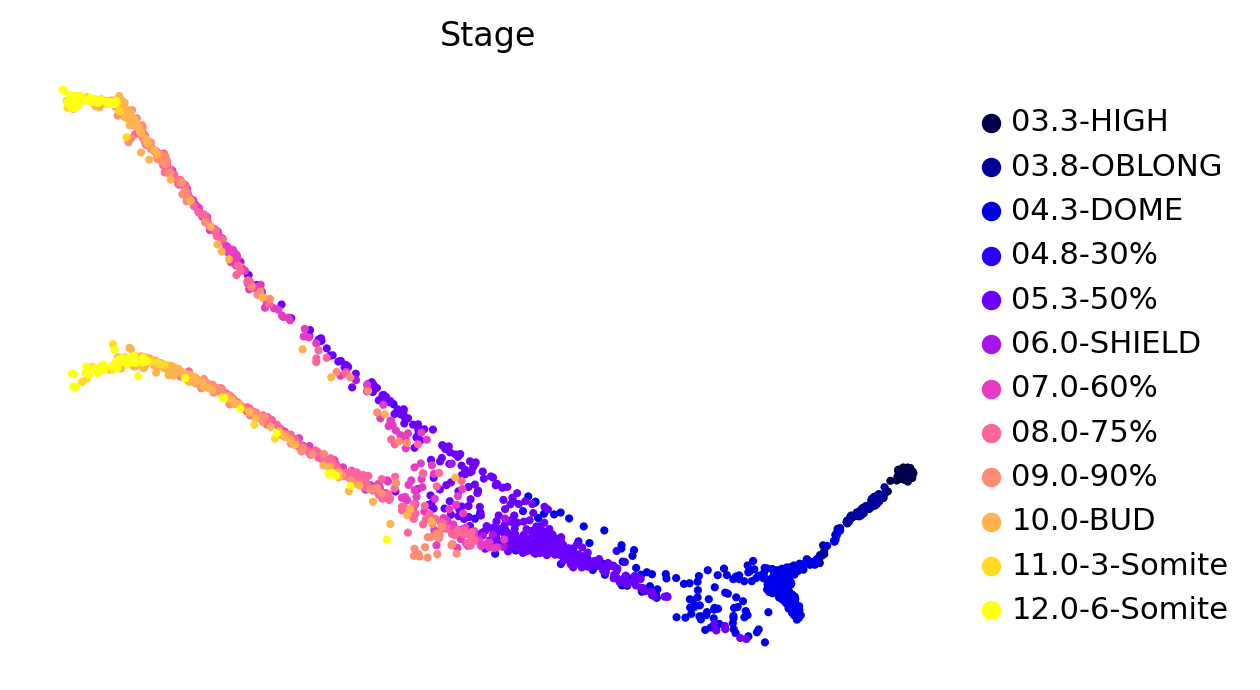We can further look into the lineage assignments, computed in the original study using URD:

In :
scv.pl.scatter(adata, basis="force_directed", c="lineages", legend_loc="right")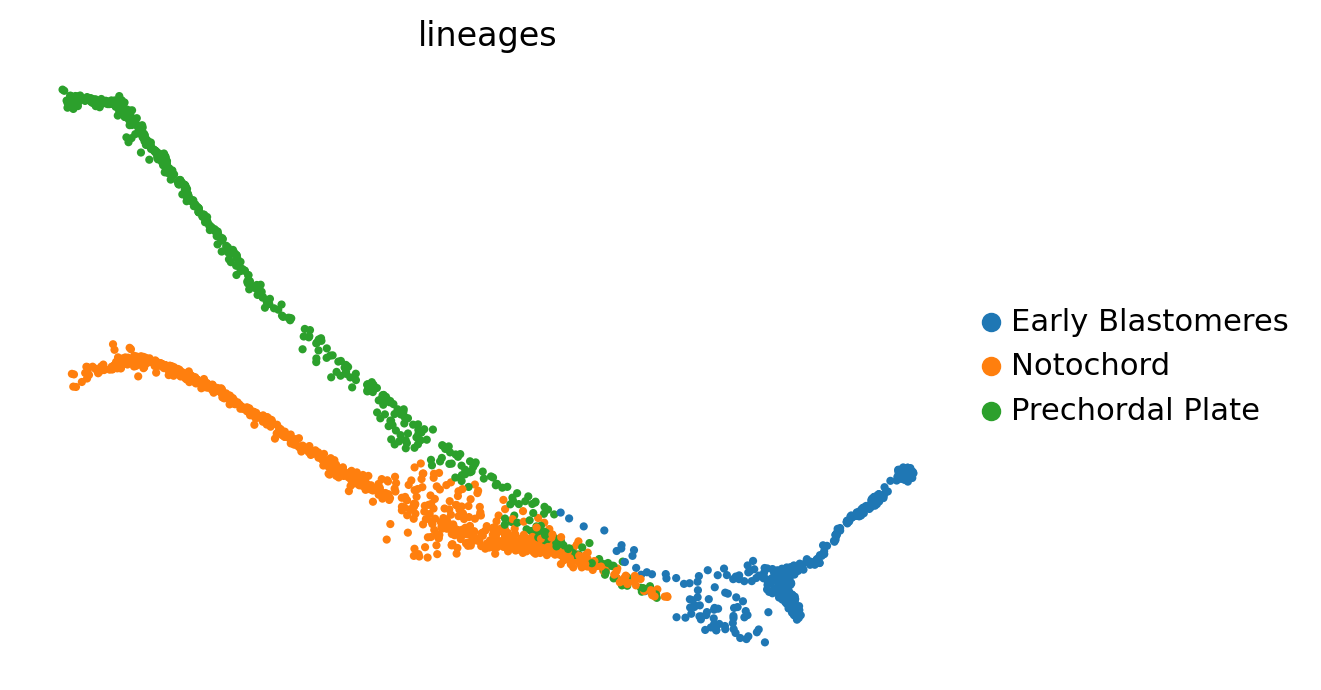## Pre-process the data¶

Before we can start applying the CytoTRACE kernel, we'll have to do some basic pre-processing of the data.

In :
# filter, normalize total counts and log-transform

# hvg annotation
print(f"This detected {np.sum(adata.var['highly_variable'])} highly variable genes. ")

# use scVelo's moments function for imputation - note that hack we're using here:
# we're copying our .X matrix into the layers because that's where scv.tl.moments
# expects to find counts for imputation

Normalized count data: X.
This detected 2392 highly variable genes.
computing neighbors
computing moments based on connectivities
'Ms' and 'Mu', moments of un/spliced abundances (adata.layers)


## Initialize the CytoTRACE kernel¶

Import the kernel and initialize it using the pre-processed AnnData object:

In :
from cellrank.tl.kernels import CytoTRACEKernel


Computing CytoTRACE score with 13690 genes
Adding adata.obs['ct_score']
adata.obs['ct_pseudotime']
adata.obs['ct_num_exp_genes']
adata.var['ct_gene_corr']
adata.var['ct_correlates']
adata.uns['ct_params']
Finish (0:00:00)


Upon initialization of the kernel, two things are computed, the actual CytoTRACE score (adata.obs['ct_score']) as well as a pseudotime (adata.obs['ct_pseudotime']) which is simply one minus the score.

Note that this is a re-implementation of the original CytoTRACE algorithm which scales better to large sample sizes because we changed the way in which gene counts are imputed. This will not exactly reproduce the original results. However, we checked in a subset of datasets from the original study that our re-implementation performs at least as good as the original implementation in terms of Spearman correlation with ground truth annotations, i.e. in capturing ground-truth differentiation status.

We can visually check that our computed CytoTRACE pseudotime correlates well with ground-truth real-time annotations:

In :
scv.pl.scatter(
c=["ct_pseudotime", "Stage"],
basis="force_directed",
legend_loc="right",
color_map="gnuplot2",
)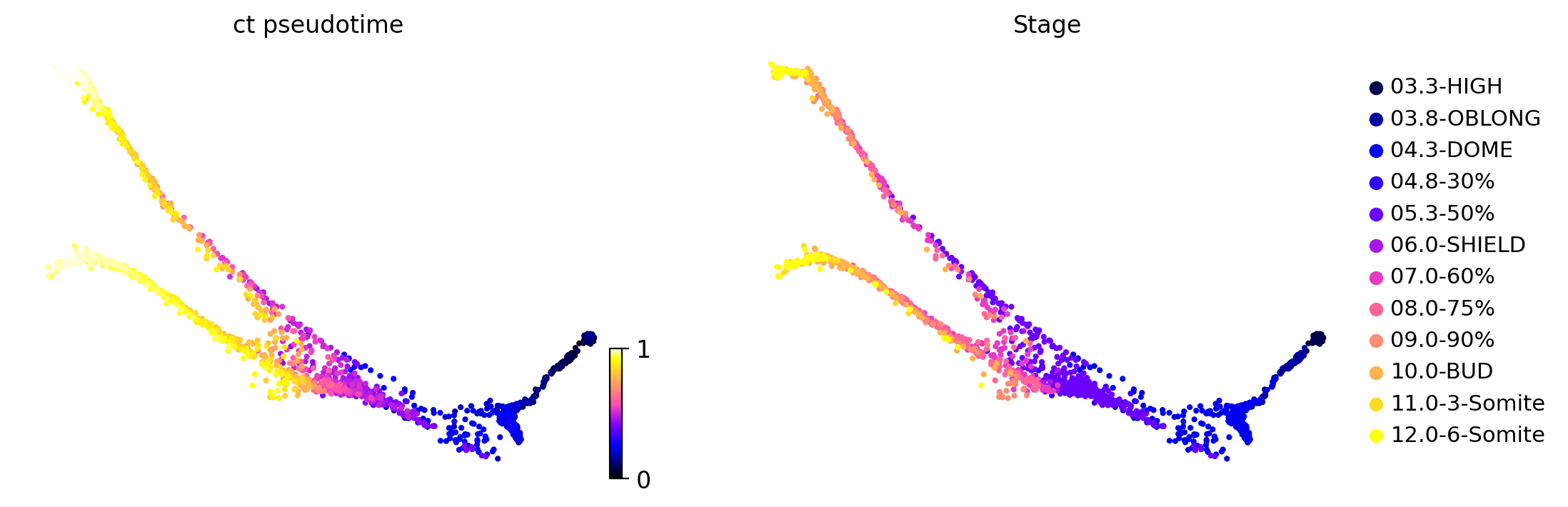To make this a bit more quantitative, we can look at the distribution of our pseudotime over real-time-points and show this via violin plots:

In :
sc.pl.violin(adata, keys=["ct_pseudotime"], groupby="Stage", rotation=90)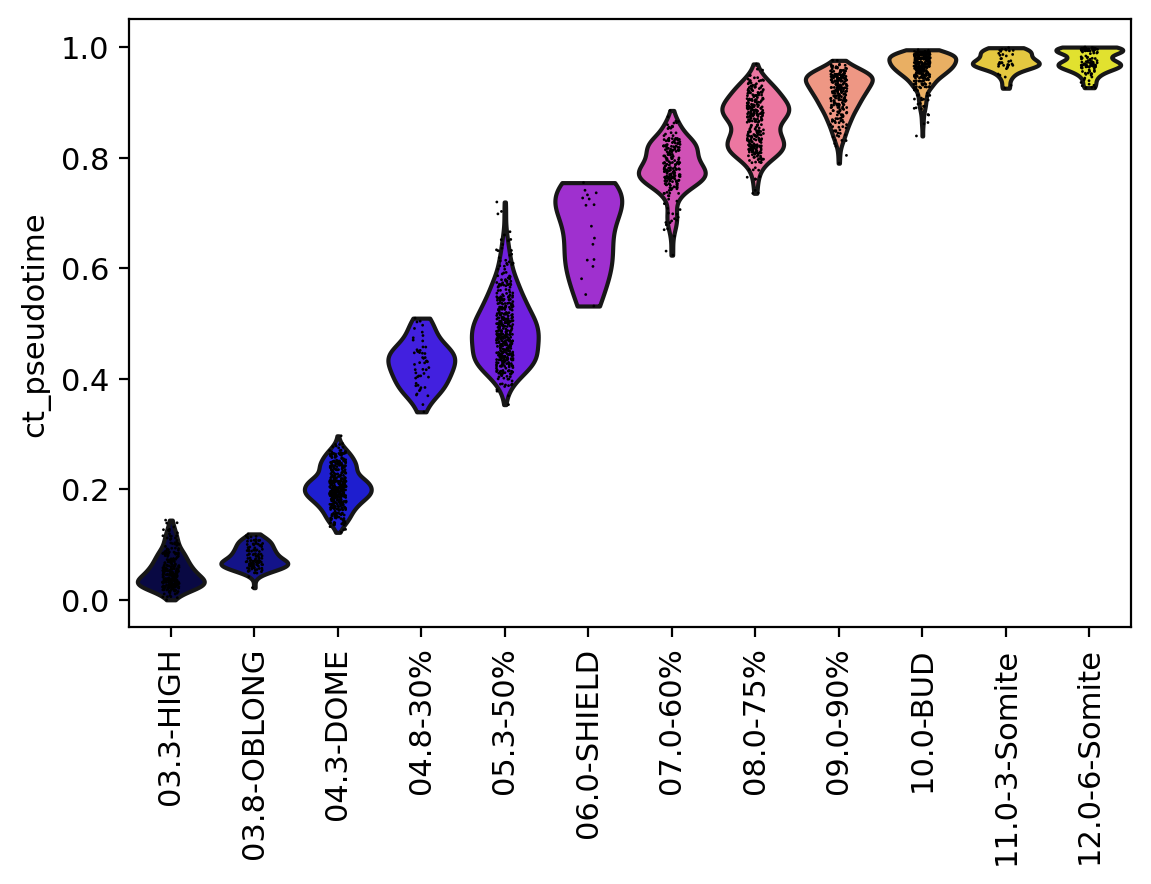The CytoTRACE pseudotime can thus be used to bias graph edges into the direction of increasing differentiation status.

## Compute & visualize a transition matrix¶

First thing we need to do is to actually compute the transition matrix:

In :
ctk.compute_transition_matrix(threshold_scheme="soft", nu=0.5)

Computing transition matrix based on ct_pseudotime
Finish (0:00:02)

100%|██████████| 2434/2434 [00:01<00:00, 2094.46cell/s]

Out:
<CytoTRACEKernel>

Under the hood, this feeds the computed CytoTRACE pseudotime into a PseudotimeKernel - our general class for taking a KNN graph and a pseudotime to compute a biased KNN graph whose edges are likely to point into the direction of increasing pseudotime. This kernel essentially combines and adapts ideas from Palantir (choose threshold_scheme='hard') and VIA (choose threshold_scheme='soft').

To get some intuition for this transition matrix, we can project it into an embedding and draw the same sort of arrows that we all got used to from RNA velocity - the following plot will look exactly like the plots you're used to from scVelo for visualizing RNA velocity, however, there's no RNA velocity here (feel free to inspect the AnnData object closely), we're visualizing the directed transition matrix we computed using the KNN graph as well as the CytoTRACE pseudotime.

In :
ctk.compute_projection(basis="force_directed")
scv.pl.velocity_embedding_stream(
)

Projecting transition matrix onto force_directed
Adding adata.obsm['T_fwd_force_directed']
Finish (0:00:00)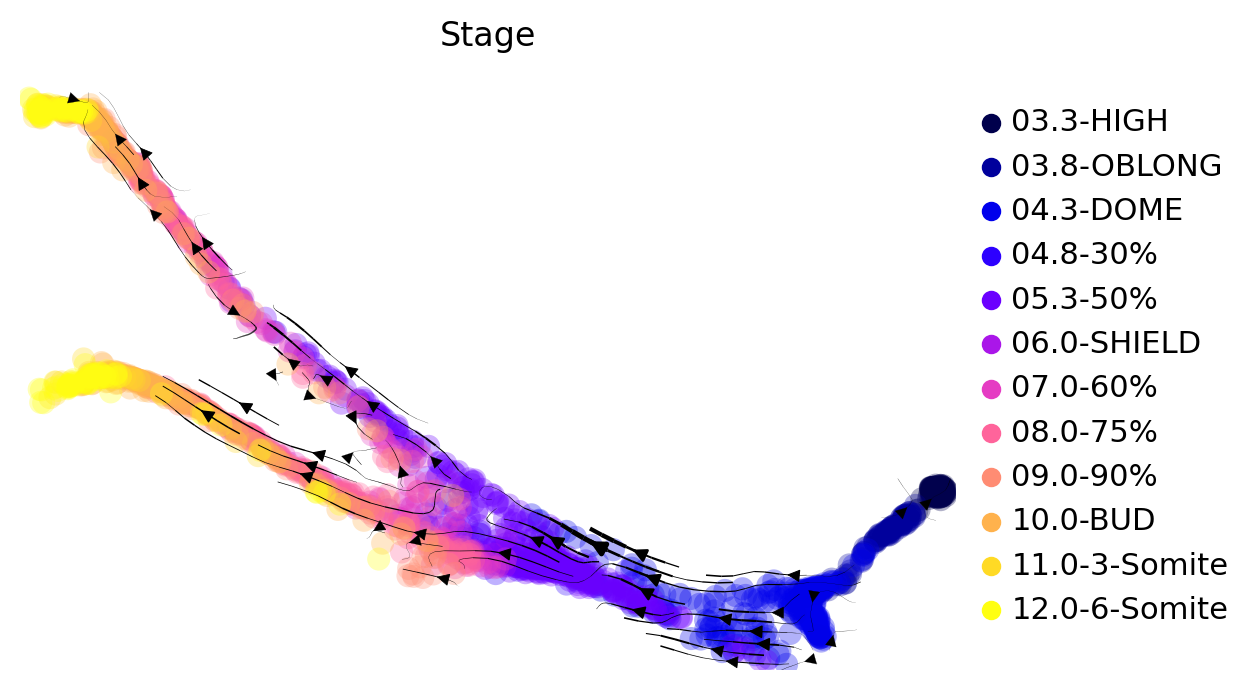The process of differentiation seems to be captured well by this transition matrix. We can visually confirm this by looking at terminal-state annotations from the original study:

In :
scv.pl.scatter(
)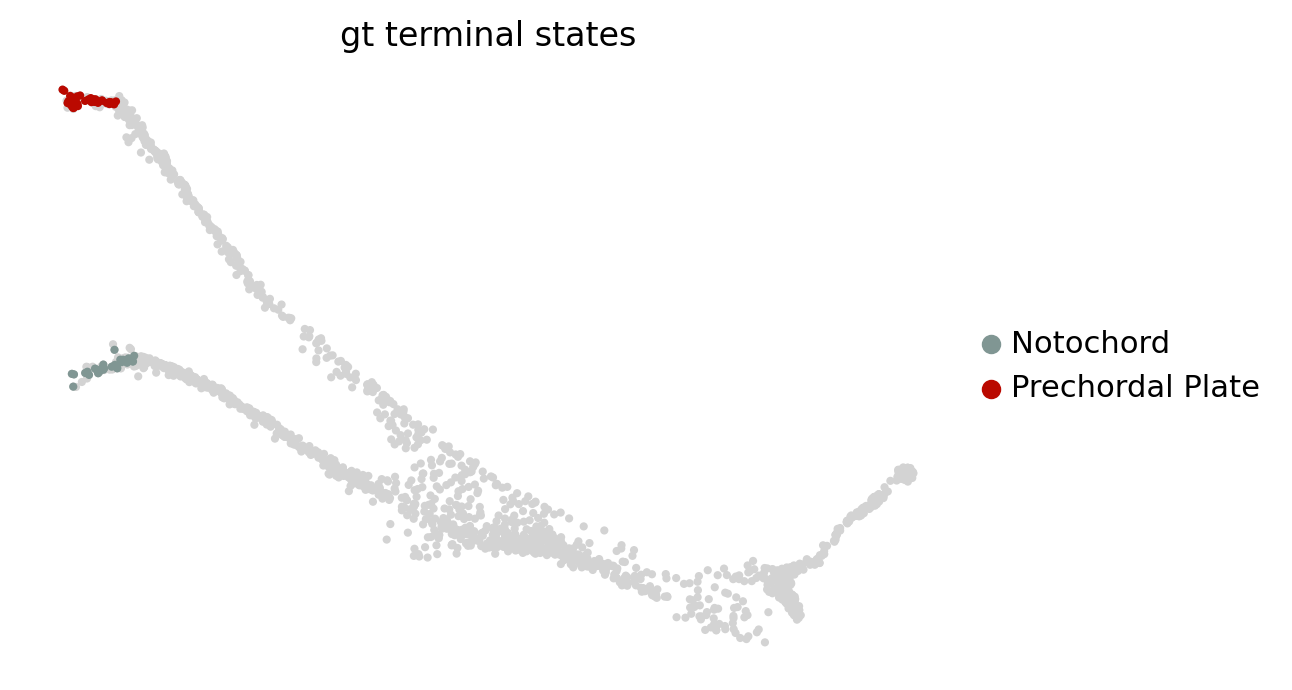Another way to gain intuition for the transition matrix is by drawing some cells from the early stage and to use these as starting cells to simulate random walks on the transition matrix:

In :
ctk.plot_random_walks(
n_sims=15,
start_ixs={"Stage": "03.3-HIGH"},
basis="force_directed",
color="Stage",
legend_loc="right",
seed=1,
)

Simulating 15 random walks of maximum length 609
Finish (0:00:02)
Plotting random walks

100%|██████████| 15/15 [00:01<00:00,  7.52sim/s]